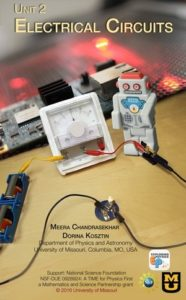### eUnit 2 Electrical CircuitsBuilding on knowledge from previous units, students will build and analyze simple circuits, and compare and contrast series and parallel circuits. Students will calculate current, voltage and resistance in a circuit. The mathematical representation of the connection between voltage, current, and resistance will be determined. By the end of the unit, students will apply their knowledge to electrical widgets that they have in their household. By the end of the unit students will be able to answer the following questions:

• What are the factors that affect the resistance of a resistor?
• How does one use current and voltage to qualitatively predict the brightness of a bulb in a circuit?
• How are voltage, current and resistance related?
• How does one describe the voltage across a resistor and the current through a resistor in a series circuit?
• How does one describe the voltage across a resistor and the current through a resistor in a parallel circuit?
• How does one calculate the power expended by a resistor?
• How does one calculate the energy expended by a resistor?

Buy materials required for teaching eUnit 2 hands-on labs.

Timeline for teaching Unit 4 (draft)

Big Ideas
1. Voltage is proportional to the electric current and the resistance of the resistor.
2. The voltage between two points in a circuit is the same for every circuit element(s) connected between the same two points (parallel circuit).
3. The current in a circuit is the same at any two points in a circuit if there are no junctions between those two points (series circuit).

Learning Goals and Objectives
By the end of this unit, the students should be able to:

1. Investigate working electrical circuits. (DOK3) a) Describe how electrical devices work. b) Use a multimeter to measure current, voltage and resistance.
2. Describe an electrical circuit, using multiple representations (i.e., pictorial diagrams, verbal descriptions, schematics, and mathematical models, etc.). (DOK3) a) Create a verbal, schematic, pictorial or mathematical description of a physical electrical circuit. b) Construct an electrical circuit from the verbal, schematic, mathematical, or pictorial representation of a circuit. c) Describe verbally and mathematically the relationship between current, voltage and resistance.
3. Design and conduct an experiment to determine how different electrodes and electrolytes affect the voltage produced by a wet-cell battery. (DOK4) a) Define voltage and measure a battery’s voltage. b) Identify the essential components of a wet-cell battery. c) Construct batteries using different combinations of electrodes and electrolytes. d) Measure the voltage of the batteries constructed.
4. Design and conduct an experiment to determine the parameters that affect the resistance of a wire. (DOK4) a) Define resistance and measure it in an electrical circuit. b) Conduct experiments to determine how the length, the diameter, and the resistivity of a wire affect its resistance and develop a mathematical relationship. c) Apply the relationship verbally and mathematically.
5. Design and conduct an experiment to determine the relationship between current, resistance and voltage within an electrical circuit. (DOK4) a) Define current and measure it in an electrical circuit. b) Design and conduct a quantitative experiment to determine a mathematical relationship among current, voltage, and resistance (Ohm’s Law, V=IR). c) Apply Ohm’s Law verbally and mathematically.
6. Mathematically, graphically and physically determine voltage, current and resistance in a series circuit. (DOK3) a) Compare and contrast the characteristics of a one-bulb circuit and a two-bulb series circuit. b) Measure the current throughout a series circuit. c) Measure the voltage of the battery and across each resistor in a series circuit and obtain a relationship among them. d) Graphically represent changes in voltage and current in a series circuit. e) Measure the equivalent resistance of a series circuit, and obtain a mathematical relationship (RS = R1 + R2 + R3 + …..) . f) Calculate the voltage, current and resistance for various series circuits. g) Calculate the voltage, current and/or resistance for various parallel circuits. h) Predict the behaviors of various mixed series/parallel circuits and short circuits.
7. Calculate the power and electrical energy used in a given electrical circuit. (DOK2) a) Relate voltage and current to power. b) Calculate the electrical power in watts, using the equation P=VI. c) Relate power to energy and time. d) Calculate the electrical energy in joules or kilowatt-hours for various circuits.
8. Design and conduct experiments to study parameters that affect electrical circuits. (DOK4) a) Make observations. b) Operationally define the variables. c) Generate a hypothesis. d) List the steps of the procedure used to collect the data. e) Collect the data and organize it into a table. f) Convert metric units as needed. g) Graph and interpret the results. h) Develop conclusions based on results.